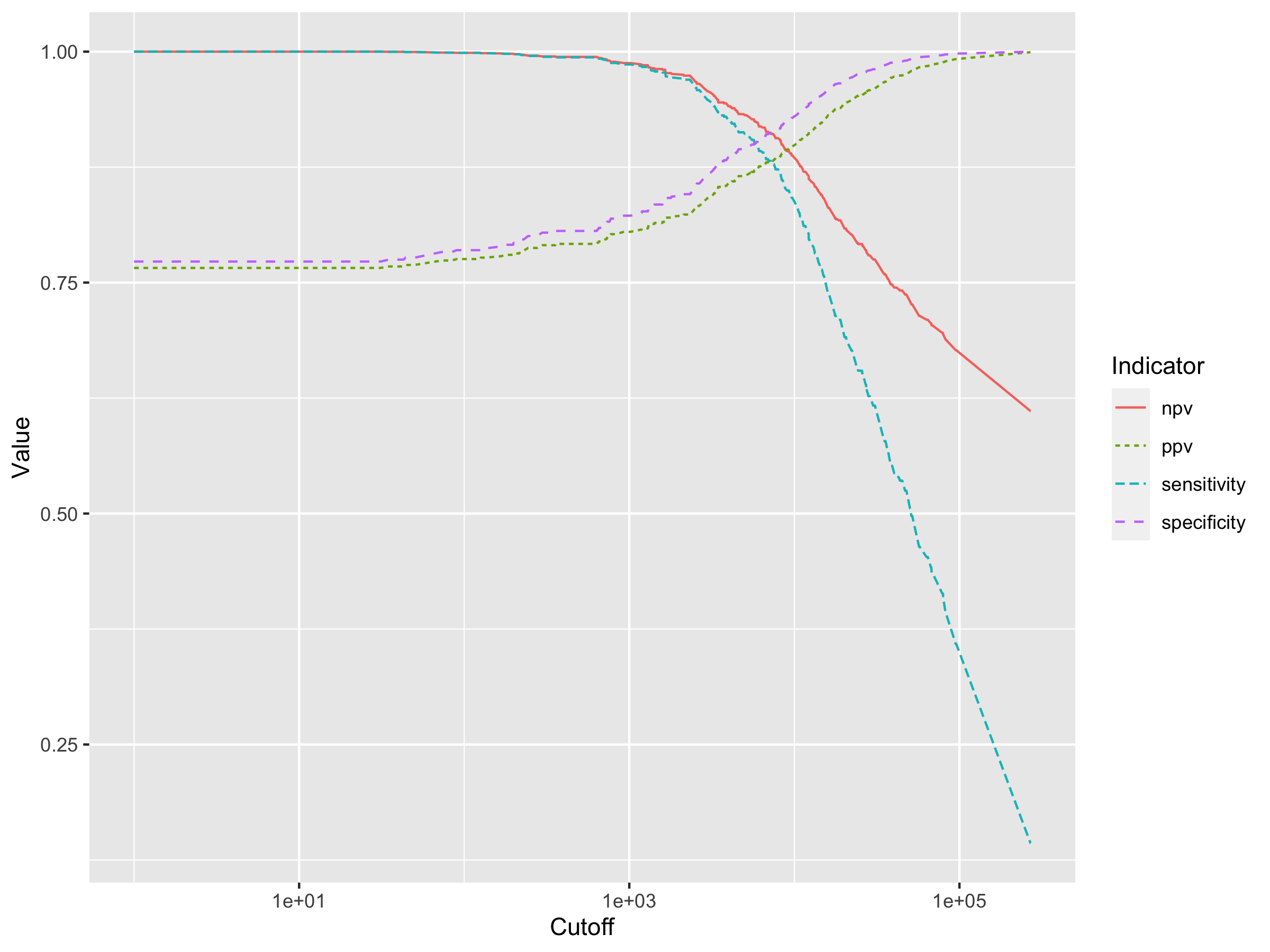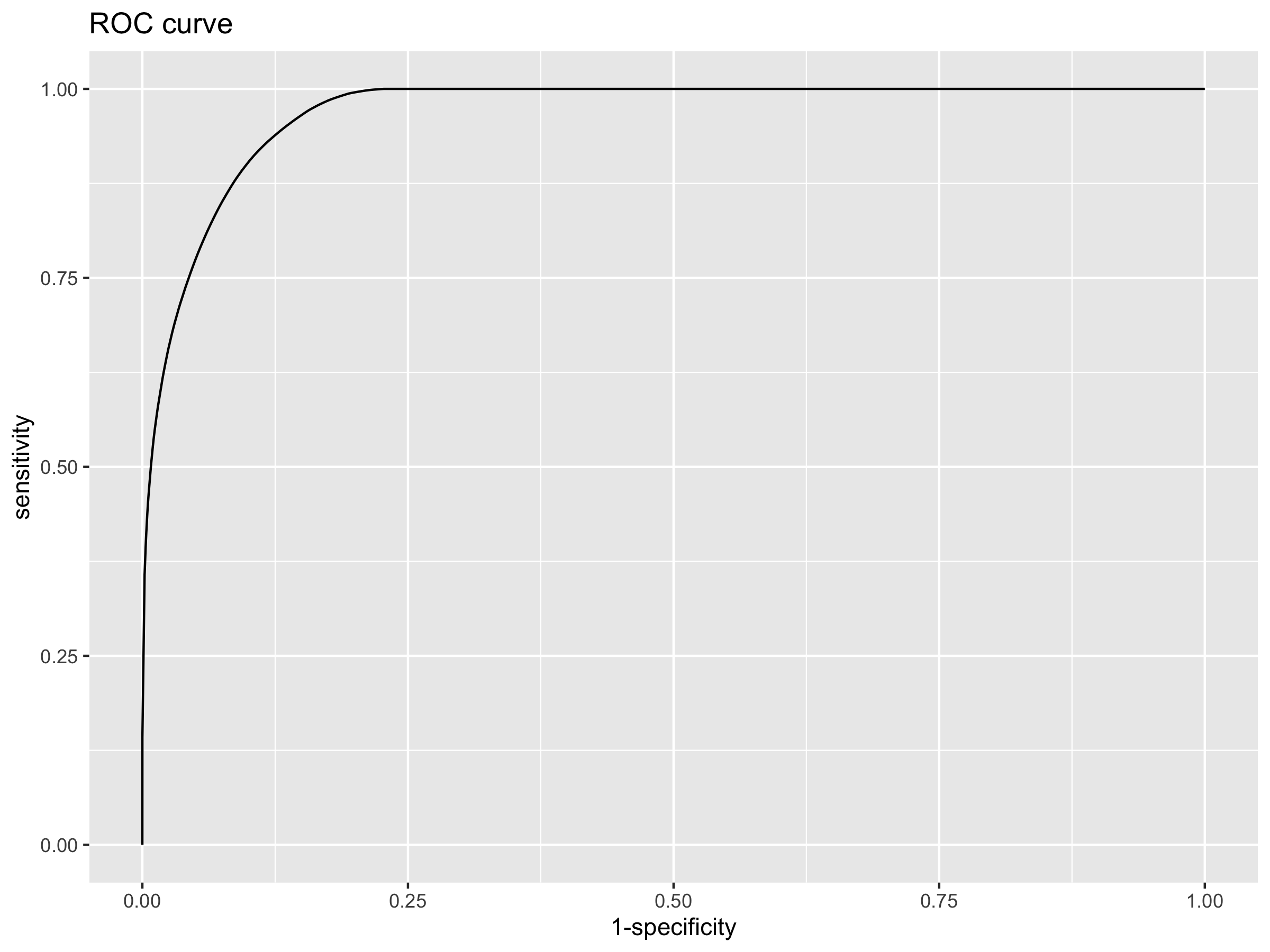# Attributable fraction using a logitexponetial model

By John Aponte and Orvalho Augusto.

## Introduction

In malaria endemic areas, asymptomatic carriage of malaria parasites occurs frequently and the detection of malaria parasites in blood films from a febrile individual does not necessarily indicate clinical malaria.

A case definition for symptomatic malaria that is used widely in endemic areas requires the presence of fever or history of fever together with a parasite density above a specific cutoff. If the parasite density is equal or higher than the cutoff point, the fever is considered due to malaria.

How to estimate what is the sensitivity and specificity of the cutoff point in the classification of the fever, without knowing what is the true value of the fevers due to malaria?

Using the attributable fraction, one can estimate the expected number of true cases due to malaria and with the positive predictive value associated with a given cutoff point, we can estimate the expected number of true cases among the fever cases that have a parasite density higher or equal than the selected cutoff point. Based on this values, the rest of the 2x2 table can be completed and the sensitivity, specificity and negative predictive value.

In order to estimate the attributable fraction and the positive predictive value, we follow the method proposed by Smith et al. (1) fitting a logistic exponential model.
Here it is presented how to do it with the afdx package for the R-software.

## Example using synthetic data

The data used in this example (malaria_df1) is a simulated data set as seen frequently in malaria cross-sectionals where two main outcomes are measured, the presence of fever or history of fever (fever column) and the measured parasite density in parasites per $$\mu l$$ (density column).

library(afdx)
#>
#> Attaching package: 'dplyr'
#> The following objects are masked from 'package:stats':
#>
#>     filter, lag
#> The following objects are masked from 'package:base':
#>
#>     intersect, setdiff, setequal, union
#>
#> Attaching package: 'magrittr'
#> The following object is masked from 'package:tidyr':
#>
#>     extract
#>
#> Attaching package: 'kableExtra'
#> The following object is masked from 'package:dplyr':
#>
#>     group_rows
head(malaria_df1) first 6 observations
fever density
1 475896
1 12008
0 1392
0 1664
0 0
1 0

In this simulation, there are 2000 observations, from which 785 have fever or history of fever and 744 have a density of malaria greater than 0. A total of 437 have both fever and a malaria density higher than 0.

Distribution of fevers by density categories
k (category lower limit) m (no fever) n (fever)
0 908 348
1 12 6
100 8 4
200 19 7
400 37 8
800 47 11
1600 43 28
3200 41 33
6400 42 51
12800 23 59
25600 24 60
51200 10 54
102400 0 50
204800 1 66

## The logit exponential model

Smith et al (1) investigate different models to describe the risk of fever due to malaria. Given that the association is not linear, the best model found was a logit exponential model:

$$logit(\pi_i) = \beta(x_i)^\tau$$

where $$\pi_i$$ is the probability of fever for the observation $$i$$ and $$x_i$$ is the parasite density for observation $$i$$.

The attributable fraction $$\lambda$$ is estimated as:

$$\lambda = (1/N)\sum{_i}{(R_i - 1)/R_i}$$

where $$N$$ is the number of fever cases, and $$R_i=exp[\beta(x_i)^\tau]$$

If a case of malaria is defined as a case of fever with a malaria density equal greater than a cutoff $$c$$, $$n_c$$ is the number of fever cases that accomplish that definition and the proportion of these diagnosis cases that are attributable to malaria $$\lambda_c$$ is estimated by

$$\lambda_c = (1/n_c)\sum{_i}d_{c,i}(R_i-1)/R_i$$

where $$d_{c,i}$$ is and indicator with value 1 if that fever case satisfies the malaria case definition and 0 otherwise

Sensitivity for the cuttof $$c$$ is defined then as: $$n_c\lambda_c / N\lambda$$,

Specificity is defined as: $$1-n_c(1-\lambda_c)/N(1-\lambda)$$

Predictive positive value as: $$n_c\lambda_c/n_c = \lambda_c$$

Negative predicted value as: $$(N(1-\lambda) - n_c(1-\lambda_c))/(N-n_c)$$

For example, if 500 is selected as cutoff point, there are 420 cases of fever with density greater or equal than 500 from a total of 785 fevers. From the model, the estimated attributable fraction is 42.63% and the proportion attributed to malaria for fevers equal or greater than 500 is 79.19%

The total number of true malaria cases is estimated as $$N\lambda=$$ 334.7 and the number of true malaria cases in those that accomplish the definition as $$n_c\lambda_c$$ = 332.6. The full 2x2 table can be filled and the corresponding sensitivity, specificity and predictive values calculated.

Case definition with 500 as cutoff
True.Malaria Other.aetiology All
Malaria case (fever and density > 500) 332.6 87.4 420
No case 2.1 362.9 365
Total 334.7 365.0 785

## Estimating the sensitivity and specificity

The adfx provide functions that facilitate the fitting of the logit exponential model and to estimate the sensitivity, specificity, positive and negative predictive values for different cutoff points.


fit <- logitexp(malaria_df1$fever, malaria_df1$density)
fit
#>              coef          se           lb          ub          z        p.val
#> alpha -0.99141023 0.061203572 -1.111367028 -0.87145343 -16.198568 5.161492e-59
#> beta   0.01057827 0.006184871 -0.001543855  0.02270039   1.710346 8.720191e-02
#> tau    0.50528526 0.054318933  0.398822110  0.61174841   9.302194 1.375767e-20
#> = = = = = = = = = =
#> AF:  0.4263407

senspec(fit, c(1,100,500,1000,2000,4000,8000,16000, 32000,54000,100000))
#>       cutoff sensitivity specificity       ppv       npv
#>  [1,]      1   1.0000000   0.7727794 0.7658523 1.0000000
#>  [2,]    100   0.9986640   0.7851103 0.7754763 0.9987369
#>  [3,]    500   0.9937947   0.8059184 0.7919064 0.9943103
#>  [4,]   1000   0.9861357   0.8224326 0.8049692 0.9876265
#>  [5,]   2000   0.9720089   0.8430225 0.8214886 0.9759178
#>  [6,]   4000   0.9250541   0.8858479 0.8576032 0.9408427
#>  [7,]   8000   0.8707223   0.9165290 0.8857481 0.9051177
#>  [8,]  16000   0.7372785   0.9594746 0.9311340 0.8309097
#>  [9,]  32000   0.5936828   0.9837722 0.9645255 0.7651378
#> [10,]  54000   0.4863329   0.9928155 0.9805100 0.7222734
#> [11,] 100000   0.3470469   0.9981097 0.9927245 0.6728613

## Graph of diagnostic characteristics for all cutoff points

The function make_cutoffs() find the densities where there is change in the number of positives and can be used to estimate the characteristics of all cutoff points

cutoffs <-  make_cutoffs(malaria_df1$fever, malaria_df1$density)
dxp <- senspec(fit, cutoffs[-1])
dxp %>%
data.frame(.) %>%
pivot_longer(-cutoff, names_to = "Indicator",values_to = "Value") %>%
ggplot(aes(x = cutoff, y = Value, color = Indicator, linetype = Indicator)) +
geom_line() +
scale_x_log10("Cutoff")## ROC curve

The receiver operative curve can be estimated from the sensitivity and specificity values

rocdf <-dxp %>%
data.frame(.) %>%
## add the corners
bind_rows(
data.frame(
sensitivity= c(1,0),
specificity= c(0,1)
)
) %>%
# generate the 1-specificity
mutate(1-specificity = 1 - specificity)

# make the graph
ggplot(rocdf, aes(x = 1-specificity, y = sensitivity)) +
geom_line()+
ggtitle("ROC curve")# Estimate the area under the curve
library(DescTools)
AUC(rocdf$1-specificity, rocdf$sensitivity)
#>  0.9695134

## Bibliography

1. Smith T, Schellenberg JA, Hayes R. Attributable fraction estimates and case definitions for malaria in endemic areas. Stat Med. 1994 Nov 30;13(22):2345–58.